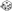General Use Thread - Page 57 - Myth-Weavers

Ootah Intimidate check:
Dice Roll: 1d20+2
d20 Results: 13 (Total = 15)

Tibbideau casts Magic Missile (sorc) at O2 (3 missiles)

Damage:
Dice Roll: 3d4+11
d4 Results: 4, 2, 4 (Total = 21)

Dice Roll: 1d20+3z
d20 Results: 13 (Total = 16)Nikhil know devotion nature (16)

Dice Roll: 1d20+10+2z
d20 Results: 11 (Total = 23)Nikhil readied attack vs W6 (23)
Dice Roll: 2d4+7+2z
d4 Results: 3, 2 (Total = 14)dmg (14)

Olbryn tries to hit Dire Wolf #2 with +1 Shock mace:
Dice Roll: 1d20+4
d20 Results: 12 (Total = 16)

If successful:
Normal damage:
Dice Roll: 1d8+1
d8 Results: 8 (Total = 9)

Electricity damage:
Dice Roll: 1d6
d6 Results: 4

Gan Power Attacks W5 with Guisarme

Hit:
Dice Roll: 1d20+8
d20 Results: 8 (Total = 16)

Damage:
Dice Roll: 2d4+17
d4 Results: 1, 4 (Total = 22)

Young Dragon

Kailus cast Magic Missile on W4

3 Magic Missiles
Damage
Dice Roll: 1D4+1z
d4 Results: 1 (Total = 2)
Dice Roll: 1D4+1z
d4 Results: 4 (Total = 5)
Dice Roll: 1D4+1z
d4 Results: 1 (Total = 2)

Dice Roll: 1d20+7+2-2z
d20 Results: 1 (Total = 8)attack W6 with PA (8)
Dice Roll: 2d4+7+2+4
d4 Results: 1, 1 (Total = 13)dmg
Dice Roll: 5d6z
d6 Results: 4, 2, 3, 1, 4 (Total = 14)Shocking grasp dmg (14)

Syndus attack
Dice Roll: 1d20+8+1z
d20 Results: 13 (Total = 22)To hit (22)
Dice Roll: 1d6+5+1z
d6 Results: 2 (Total = 8)dmg (8)

Ootah tries to break grapple against Owlbear 1:
Dice Roll: 1d20+6
d20 Results: 2 (Total = 8)